# GSEB Solutions Class 11 Maths Chapter 9 Sequences and Series Ex 9.2

Gujarat Board GSEB Textbook Solutions Class 11 Maths Chapter 9 Sequences and Series Ex 9.2 Textbook Questions and Answers.

## Gujarat Board Textbook Solutions Class 11 Maths Chapter 9 Sequences and Series Ex 9.2

Question 1.
Find the sum of odd integers from 1 to 2001.
Solution:
We have to find the sum 1 + 3 + 5 + ………….. + 2001.
Let the nth term be the last term.
∴ a + (n – 1)d = 2001.
Here, a = 1, d = 2.
∴ 1 + [n – 1].2 = 2001 or 2[n – 1] = 2000 – 1 = 2000
∴ n – 1 = 2000, n = 1001
∴ Sum = $$\frac{n}{2}$$ [2a + (n – 1)d]
= $$\frac{1001}{2}$$[2.1 + (1001 – 1) × 2]
= $$\frac{1001}{2}$$ × 2[1 + 100 – 1] = 1001 × 1001
= 1002001.Question 2.
Find the sum of all natural numbers lying between 100 and 1000 which are multiples of 5?
Solution:
We have to find the sum 105 + 110 + 115 + … 995
Let 995 = nth term.
∴ a + (n – 1)d = 995.
n = 199 – 20 = 179
∴ 105 + 110 + 115 + ……….. + 995 = $$\frac{n}{2}$$[2a + (n – 1)d]
= $$\frac{179}{2}$$ [2 × 105 + (179 – 1) × 5]
= $$\frac{179}{2}$$ [2 × 105 + 5 × 178]
= 179(105 + 5 × 89)
= 179(105 + 445) = 179 × 550
= 98450.Question 3.
In an A.P., the first term is 2 and the sum of first five terms is one-fourth of the sum of next five terms. Show that its 20th term is – 112.
Solution:
Let d be the common difference.
∴ Sum of first 5 terms = $$\frac{n}{2}$$ [(2a + (n – 1))d]
= $$\frac{5}{2}$$ [2 × 2 + (5 – 1)d]
= $$\frac{5}{2}$$[4 + 4d] = 5(2 + 2d).
6thterm = a + (6 – 1)d = a + 5d.
∴ (a + 5d) + (a + 6d) + ………….. to 5 terms
= $$\frac{5}{2}$$ [2(a + 5d) + (5 – 1)d]
= $$\frac{5}{2}$$ [2a + 10d + 4d]
= $$\frac{5}{2}$$ [2a + 14d] = 5(a + 7d)
= 5(2 + 7d)
Sum of first five terms = $$\frac{1}{4}$$ × sum of next 5 terms
5(2 + 2d) = $$\frac{1}{4}$$ × 5(2 + 7d)
or 4(2 + 2d) = 2 + 7d
∴ d = – 6
∴ T20 = a + (20 – 1)d = 2 + 19 × (- 6)
= 2 + (- 114) = – 112.4. How many terms of the A.P. – 6, – $$\frac{11}{2}$$, – 5, ……………… are needed to give the sum – 25?
Solution:
Here, a = – 6, d = – $$\frac{11}{2}$$ – (- 6) = $$\frac{- 11}{2}$$, – 5, …………….. are needed to give the sum – 25?
Solution:
Here, a = – 6, d = – $$\frac{11}{2}$$ – (- 6) = $$\frac{- 11}{2}$$ + 6 = $$\frac{- 11+12}{2}$$ = $$\frac{1}{2}$$.
Let n be the number of terms, so that
Sn = – 25.
Since Sn = $$\frac{n}{2}$$[2a + (n – 1)d], we have: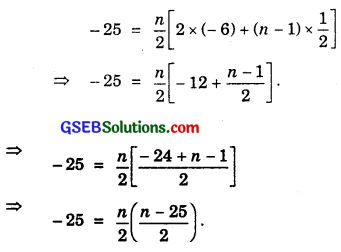⇒ – 100 = n(n – 25)
⇒ n2 – 25n + 100 = 0
⇒ (n – 5)(n – 20) = 0
⇒ n = 5 or n = 20.
Both values of n give the required sum.

Question 5.
In an A.P., if the pth term is $$\frac{1}{q}$$ and the qth term is $$\frac{1}{p}$$, prove that the sum of first pq terms is $$\frac{1}{2}$$(pq + 1), where p ≠ q?
Solution:
Let a be the first term and d be the common difference of £he A.P. Then,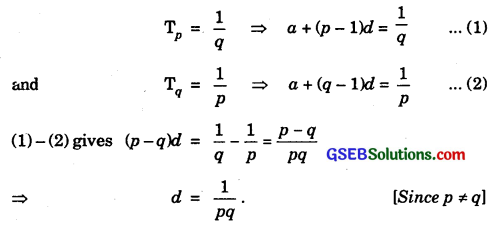Putting d = $$\frac{1}{pq}$$ in (1), we get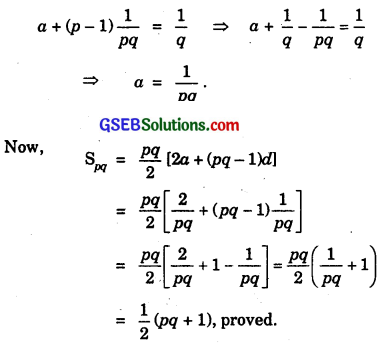Question 6.
If the sum of a certain number of terms of the A.P; 25, 22, 19, ………………. is 116. Find the last term?
Solution:
a = 25, d = 22 – 25 = – 3. Let n be the number of terms.
Sum = 116.
Now, sum = $$\frac{n}{2}$$ [2a + (n – 1)d]
∴ 116 = $$\frac{n}{2}$$ [50 + (n – 1)(- 3)]
or 232 = n[50 – 3n + 3] = n[53 – 3n]
= – 3n2 + 53n.
⇒ 3n2 – 53n + 232 = 0.
⇒ (n – 8)(3n – 29) = 0.
∴ n = 8 or $$\frac{29}{3}$$. But n ≠ $$\frac{29}{3}$$.
∴ n = 8.
Now, T8 = a + (8 – 1)d = 25 + 7 × (- 3)
= 25 – 21 = 4.
∴ Last term = 4.

Question 7.
Find the sum to n terms of an A.P., whose kth term is 5k + 1.
Solution:
Tk = 5k + 1.
Putting k = 1 and 2, we get
T1 = 5 × 1 + 1 = 5 + 1 = 6
and T2 = 5 × 2 + 1 = 10 + 1 = 11.
∴ d = T2 – T1 = 11 – 6 = 5.
Here, a = 6, d = 5.
Sum of n terms = $$\frac{n}{2}$$[2a + (n – 1)d].
= $$\frac{n}{2}$$[2 × 6 + (n – 1) × 5]
= $$\frac{n}{2}$$[12 + 5n – 5]
= $$\frac{n(5n+7)}{2}$$.Question 8.
If the sum to n terms of an A.P. is (pn + qn2), where p and q are constants, find the common difference?
Solution:
Let Sn be the sum to n terms.
∴ Sn = pn + qn2
Putting n = 1 and 2, we get
S1 = T1 = p.1 + q.12 = p + q
and S2 = T1 + T2 = p.2 + q.22 = 2p + 4q.
T2 = (T1 + T2) – T1 = S2 – S1
= [(2p + 4q)] – (p + q)]
= p + 3q.
∴ d = T2 – T1 = (p + 3q) – (p + q) = 2q.
∴ Common differences of the A.P. is 2q.

Question 9.
The sums of first n terms of two arithmetic progressions are in the ratio (5n + 4) : (9n + 6). Find the ratio of their 18th terms?
Solution:
Let a1 and d1 be the first term and common difference of the first A.P. and a2 and d2 be the first term and common difference of the second A.P.
If S1 and S2 be their sums respectively, then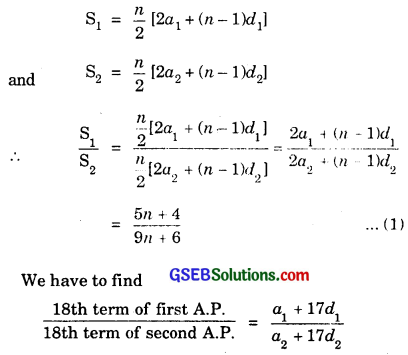Multiplying numerator and denominator by 2, we get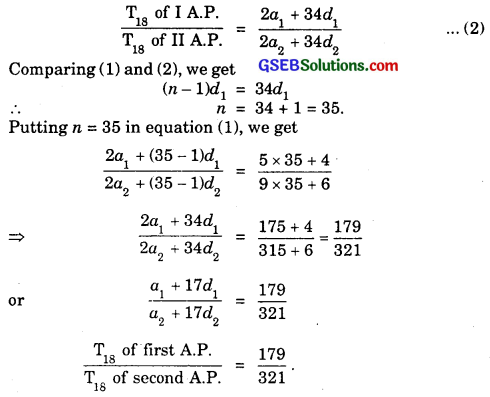Question 10.
If the sum of first p terms of an A.P. is equal to the sum of first q terms, then find the sum of first (p + q) terms?
Solution:
Let a be the first term and d be the common difference of A.P.
∴ Sum of first p terms = $$\frac{p}{2}$$[2a + (p – 1)d] ……………. (1)
and sum of first q terms = $$\frac{q}{2}$$[2a + (q – 1)d] …………….. (2)
Equating (1) and (2), we get
$$\frac{p}{2}$$[(2a + (p – 1)d] = $$\frac{q}{2}$$[2a + (q – 1)d].
Transposing the terms of R.H.S. to L.H.S., we get
2a(p – q) + [p(p – 1) – q(q – 1)d] = 0
or 2a(p – q) + [p2 – q2 – (p – q)d] = 0
or 2a(p – q) + (p – q)[(p + q) – d] = 0
or (p – q)[2a + (p + q – 1)d] = 0
⇒ 2a + (p + q – 1)d = 0
Sum of first (p + q) terms = $$\frac{p+q}{2}$$[2a + (p + q – 1)d]
= $$\frac{p+q}{2}$$ × 0 = 0
[∵ 2a + (p + q – 1)d = 0 from (3)]

Question 11.
Sum of the first p, q and r terms of an A.P. are a, b and c respectively. Prove that $$\frac{a}{p}$$(q – r) + $$\frac{b}{q}$$(r – p) + $$\frac{c}{r}$$(p – q) = 0.
Solution:
Let A be the first term and D be the common difference of A.P.
Sp = a.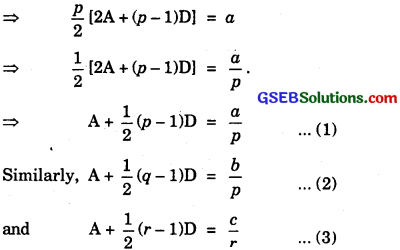Multiplying (1) by (q – r), (2) by (r – p), (3) by (p – q) and adding we get
A(q – r + r – p + p – q) + $$\frac{1}{2}$$[(p – 1)(q – r)(r – q) + (r – 1)(p – q)]D
= $$\frac{a}{p}$$(q – r) + $$\frac{b}{q}$$(r – p) + $$\frac{c}{r}$$(p – r)
⇒ A(0) + $$\frac{1}{2}$$(0)D = $$\frac{a}{p}$$(q – r) + $$\frac{b}{q}$$(r – p) + $$\frac{c}{r}$$(p – r).
Hence, $$\frac{a}{p}$$(q – r) + $$\frac{b}{q}$$(r – p) + $$\frac{c}{r}$$(p – q) = 0.Question 12.
The ratio of the sums of first m and the terms of an A.P. is m2 : n2. Show that the ratio of mth and nth terms is 2m – 1 : 2n – 1.
Solution:
Let a be the first term and d be the common difference of A.P.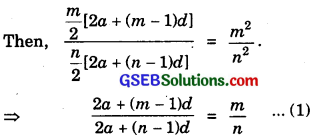i.e; We are to find the value of $$\frac{a+(m – 1)d}{a+(n – 1)d}$$ = $$\frac{2a+(2m-2)d}{2a+(2n-2)d}$$
L.H.S. of (1) will be identical with (2), if (m – 1) replaces (2m – 2) and (n – 1) replaces (2n – 2). So, replacing m by 2m – 1 and n by 2n – 1 on both sides of (1), we get
$$\frac{2a+(2m-2)d}{2a+(2n-2)d}$$ = $$\frac{(2m-1)}{(2n-1)}$$.

Question 13.
If the sum of first re terms of an A.P. is 3n2 + 5n and its mth term is 164, find the value of m?
Solution:
Let the sum of first re terms be denoted by Sp.
∴ Sn = 3n2 + 5n.
Put n = 1 and 2.
T1 = S1 = 3.12 + 5.1 = 3 + 5 = 8.
S2 = T1 + T2 = 3.22 + 5.2 = 12 + 10 = 22.
∴ T2 = S2 – S1 = 22 – 8 = 14.
∴ Common difference d = T2 – T1 = 14 – 8 = 6.
a = 8, d = 6.
mth term = a + (m – 1)d = 164.
or 8 + (m – 1)6 = 164.
⇒ 6m + 2 = 164 or 6m = 164 – 2 = 162.
∴ m = $$\frac{162}{6}$$ = 27.Question 14.
Insert five numbers between 8 and 26 such that the resulting sequence is an A.P.
Solution:
Let the five numbers be denoted by T2, T3, T4, T5 and T6.
∴ 8, T2, T3, T4, T5, T6 and 26 are in A.P.
26 is the 7th term. If d is the common difference,
then T7 = a + (7 – 1)d.
⇒ 26 = 8 + 6d ⇒ 6d = 26 – 8 = 18
∴ d = 3.
So, T2 = a + d = 8 + 3 = 11,
T3 = a + 2d = 8 + 16 = 14,
T4 = a + 3d = 8 + 9 = 17,
T5 = a + 4d = 8 + 12 = 20,
and T6 = a + 5d = 8 + 15 = 23.
Required five numbers are 11, 14, 17, 20 and 23.

Question 15.
If $$\frac{a^{n}+b^{n}}{a^{n-1}+b^{n-1}}$$ is the A.M. between a and b, then find the value of n?
Solution:
A.M. between a and b = $$\frac{a+b}{2}$$
∴ $$\frac{a^{n}+b^{n}}{a^{n-1}+b^{n-1}}$$ = $$\frac{a+b}{2}$$
⇒ 2an + 2bn = an + abn-1 + an-1b + bn
⇒ an – an-1b – abn-1 + bn = 0
⇒ an-1(a – b) – bn-1(a – b) = 0
⇒ (a – b)(an-1 – bn-1) = 0 [∵a ≠ 0]
⇒ an-1 – bn-1 = 0
⇒ an-1 = bn-1.
⇒ $$\frac{a}{b}$$n-1 = 1 = ($$\frac{a}{b}$$)0
⇒ n – 1 = 0 ⇒ n = 1.Question 16.
Between 1 and 31, m numbers have been inserted in such a way that the resulting sequence is an A.P. and the ratio of 7th and (m – 1)th numbers is 5:9. Find the value of m?
Solution:
Let A1, A2, ……………….. Am be the m arithmetic means each inserted between 1 and 31.
Total number of terms = m + 2.
If d is the common difference, then
1 + (m + 2 – 1 )d = 31.
⇒ (m + 1)d – 30.
⇒ d = $$\frac{30}{m+1}$$.
So, A7 = T8 = a + 7d
= 1 + 7 × $$\frac{30}{m+1}$$
= $$\frac{m+1+210}{m+1}$$ = $$\frac{m+211}{m+1}$$
Also, Am-1 = Tm = a + (m – 1)d
= 1 + (m – 1) × $$\frac{30}{m+1}$$
= $$\frac{m+1+30m-30}{m+1}$$ = $$\frac{31m-29}{m+1}$$
Now, $$\frac{A_{7}}{A_{m-1}}$$ = $$\frac{m+211}{31m-29}$$ = $$\frac{5}{9}$$.
⇒ 9m + 1899 = 155m – 145
⇒ 146m = 2044
⇒ m = $$\frac{2044}{146}$$ = 14
Hence, m = 14.Question 17.
A man starts repaying a loan as first instalment of ₹ 100. If he increases the instalment by ₹ 5 every month, what amount he will pay in the 30th instalment?
Solution:
The instalments form an A.P., whose first term is ₹ 100 and the common difference is ₹ 5.
∴ 30th instalment = T30 = a + (30 – 1)d
= 100 + 29 × 5
= 100 + 143
= 245.
Thus, the 30th instalment is ₹ 245.Question 18.
The difference between any two consecutive interior angles of a polygon is 5°. If the smallest angle is 120°, find the number of sides of the polygon?
Solution:
The angles of a polygon of n sides form an A.P., whose first term is 120° and common difference is 5°.
The sum of interior angles (in degrees)
= $$\frac{n}{2}$$ [2a + (n – 1)d]
= $$\frac{n}{2}$$ [2 × 120 + (n – 1) × 5]
= $$\frac{n}{2}$$ [240 + 5n – 5] = $$\frac{n}{2}$$(235 + 5n).
Also, the sum of interior angles (in degrees)
= 180 × n – 360.
∴ $$\frac{n}{2}$$(235 + 5n) = 180n – 360
Multiplying by $$\frac{2}{5}$$, we get
n(47 + n) = 2(36n – 72)
= 72n – 144
or n2 + (47 – 72)n + 144 = 0.
⇒ n2 – 25n + 144 = 0
⇒ (n – 16)(n – 9) = 0
Now, n ≠ 16
∴ n = 9.